Courses

# Test: Fractions - 2

## 20 Questions MCQ Test Mathematics (Maths) Class 6 | Test: Fractions - 2

Description
This mock test of Test: Fractions - 2 for Class 6 helps you for every Class 6 entrance exam. This contains 20 Multiple Choice Questions for Class 6 Test: Fractions - 2 (mcq) to study with solutions a complete question bank. The solved questions answers in this Test: Fractions - 2 quiz give you a good mix of easy questions and tough questions. Class 6 students definitely take this Test: Fractions - 2 exercise for a better result in the exam. You can find other Test: Fractions - 2 extra questions, long questions & short questions for Class 6 on EduRev as well by searching above.
QUESTION: 1

Solution:
QUESTION: 2

### A two-digit number is such that the product of the digits is 8. When 18 is added to the number, then the digits are reversed. The number is:

Solution:

Let the ten's and unit digit be x and 8/x respectively.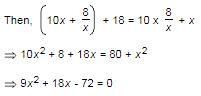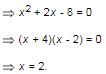QUESTION: 3

### What fraction of these circles have X's in them?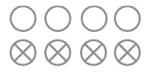Solution:
QUESTION: 4

Write the fraction representing the shaded region in the below figure.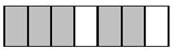Solution:
QUESTION: 5

What is the fraction of the shaded area?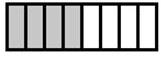Solution:
QUESTION: 6

What is the fractional form of five eighteenths?

Solution:

The fractional form of five eighteenths

= 5/18

QUESTION: 7

The simplest form of 16/72 is ________

Solution:
QUESTION: 8

What fraction of a day is 8 hours?

Solution:
QUESTION: 9

Express as improper fraction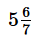Solution:
QUESTION: 10

Write the fraction representing the shaded portion.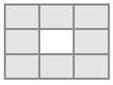Solution:
QUESTION: 11

Fractions with same denominators are called ________.

Solution:

Like Fractions: The fractions having the same denominators are known as like fractions. We know that fractions such as 2/5, 3/5, 5/5, 7/5, 9/5, …. etc., are like fractions.

QUESTION: 12

Express as mixed fraction 20/3.

Solution:
QUESTION: 13

The simplest form of 15/75 is ________.

Solution:
QUESTION: 14

What fraction of an hour is 40 minutes?

Solution:
QUESTION: 15

Give a proper fraction whose denominator is 7 and numerator is

Solution:
QUESTION: 16

In a proper fraction the ___________ shows the number of parts which have been considered.

Solution:
QUESTION: 17

Write the natural numbers from 102 to 113. What fraction of them are prime numbers?

Solution:

Natural numbers from 102 to 113: 102, 103, 104, 105, 106, 107, 108, 109, 110, 111, 112, 113
Prime numbers from 102 to 113: 103, 107, 109, 113
Hence fraction of prime numbers = 4/12 = 1/3

QUESTION: 18

A _________ means a part of a group or of a region.

Solution:
QUESTION: 19

Fractions with not same denominators are called ________.

Solution:
QUESTION: 20

Write the fraction representing the shaded region in the below figure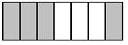Solution: